Q.1
• E/2
• 4E/3
• √ 2 E
• 3E/4
Q.2
##### Total energy of mass spring system in harmonic motion is E=1/2(mω2A2). Consider another system executing SHM with same amplitude having value of spring constant as half the previous one and mass twice as that of previous one. The energy of second oscillator will be
• E
• 2E
• $\sqrt 2 E$
• E/2
Q.3
• √(k/mr)
• √(kr/m)
• √(k/m)
• √(2k/m)
Q.4
##### The total energy of the particle executing SHM  is Here x is the displacement of the particle
• $\alpha x^2$
• $\alpha x$
• $\alpha x^{1/2}$
• Independent of x
Q.5
##### In a spring mass system executing SHM having mass m and spring constant K with Time period T. Match the column I to the column II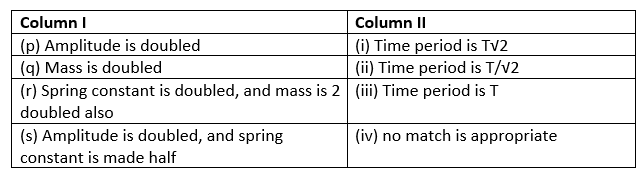• p-> iv, q -> i ,r -> iii, s-> ii
• p-> iii, q -> i ,r -> iv, s-> ii
• p-> i, q -> i ,r -> iii, s-> ii
• p-> iii, q -> i ,r -> iii, s-> ii
Q.6
##### If a simple harmonic motion is represented by $\frac {d^2x}{dt^2} + \beta x=0$, then its time period is
• $\frac {2 \pi}{\sqrt {\beta}}$
• $\frac {2 \pi}{\beta}$
• $2 \pi \beta$
• $2 \pi\sqrt {\beta}$
Q.7
• 3/2
• 1/16
• 1/4
• 1/2√ 2
Q.8
##### In the system shown below frequency of oscillation when mass is displaced slightly is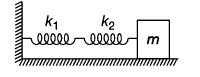• $f=\frac {1}{2\pi} \sqrt { \frac {k_1k_2}{k_1+k_2)m}}$
• $f=\frac {1}{2\pi} \sqrt { \frac {k_1+k_2}{m}}$
• $f=\frac {1}{2\pi} \sqrt { \frac {m}{k_1 k_2}}$
• $f=\frac {1}{2\pi} \sqrt { \frac {k_1+k_2}{mk_1 k_2}}$
Q.9
##### find the maximum static frictional force on the body M2
• $\mu _s g M_1$
• $\mu _s g M_2$
• $\mu _s g (M_1 + M_2)$
• None of these
Q.10
##### A solid cylinder of mass m is attached to a horizontal spring with force constant k. The cylinder can roll without slipping along the horizontal plane. Center of mass of the cylinder executes simple harmonic motion if displaced from its mean position,The time period is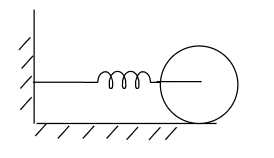• $T= 2 \pi \sqrt { \frac {5m}{k}}$
• $T= 2 \pi \sqrt { \frac {3m}{2k}}$
• $T= 2 \pi \sqrt { \frac {m}{3k}}$
• $T= 2 \pi \sqrt { \frac {m}{k}}$
Q.11
##### Which one of the following statements is true for the speed v and the acceleration a of a particle executing simple harmonic motion?
• When v is maximum, a is maximum
• Value of a is zero, whatever may be the value of v.
• When v is maximum, a is zero.
• When v is zero, a is zero
Q.12
##### Find the maximum oscillation magnitude Amax that permits the two bodies to move as unit
• $A_{max}=\frac {(1+a)\mu _s gM_1}{k}$
• $A_{max}=\frac {\mu _s gM_1}{k}$
• $A_{max}=\frac {\mu _s gM_2}{k}$
• none of the above
Q.13
• .1m/s
• 5.0m/s
• 1m/s
• 1.5m/s
Q.14
• T
• 2T
• T/2
• T/4
Q.15
• 4k/5
• 5k/4
• 3k/2
• 4k
Q.16
• 1:2√ 2
• 2√ 2:1
• 1:√ 2
• √ 2:1
Q.17
##### Fig below shows two equal masses of mass m joined by a rope passing over a light pulley. First mass is attached to a spring and another end of spring is attached to a rigid support. Neglecting frictional forces total energy of the system when spring is extended by a distance x is Here, v = dx/dt , the velocity of mass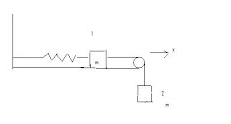• mv2+1/2(Kx2)+mgx
• mv2-1/2(Kx2)+mgx
• mv2-1/2(Kx2)-mgx
• mv2+1/2(Kx2)-mgx
Q.18
##### A block of mass M1 resting on the frictionless surface is connected to a spring of spring constant k that is anchored in the nearby wall. A block of mass M2=aM1 is placed on the top of the first block. The coefficient of static friction between the two bodies is μs Assuming the two bodies moves as single object, find the period of oscillation of the system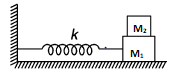• $T=2 \pi \sqrt {\frac {(1+a)M_1}{k}}$
• $T=2 \pi \sqrt {\frac {M_1}{k}}$
• $T=2 \pi \sqrt {\frac {(1-a)M_1}{k}}$
• $T=2 \pi \sqrt {\frac {aM_1}{k}}$